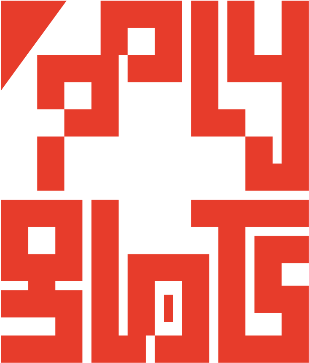# polygl0ts

### EPFL's CTF team# TUCTF 2018: AESential Lesson

## Description

Thought I’d give you an essential lesson to how you shouldn’t get input for AES in ECB mode.

nc 18.218.238.95 12345

The server run the python file `redacted.py`

``````#!/usr/bin/env python2

from Crypto.Cipher import AES

from select import select

import sys

INTRO = """
Lol. You think you can steal my flag?
I\'ll even encrypt your input for you,
but you can\'t get my secrets!

"""

flag = "REDACTED" # TODO Redact this

key = "REDACTED" # TODO Redact this

if __name__ == '__main__':

padc = 'REDACTED' #TODO Redact this

assert (len(flag) == 32) and (len(key) == 32)

cipher = AES.new(key, AES.MODE_ECB)

sys.stdout.write(INTRO)
sys.stdout.flush()

while True:
try:
sys.stdout.flush()

rlist, _, _ = select([sys.stdin], [], [])

inp = ''
if rlist:

plaintext = inp + flag
l = len(plaintext)

padl = (l // 32 + 1)*32 if l % 32 != 0 else 0

except KeyboardInterrupt:
exit(0)
``````

## Solution

### Analysis of the code

• First of all we see that the challenge is based on AES in ECB mode. It is highly insecure when encrypting over multiple blocks. One of the reason is that we can differentiate text based of their ciphertext. This means that if we encrypt:

block 1, block 2, block 3 `aaaa....aaaa, bbbb....bbbb, aaaa....aaaa`

the ciphertext of the block 1 will be equals to the one in the block 3.

• In the code the flag size is constant to 32 characters, the exact size of a block.

• We can concatenate text on the left side of the flag before encryption.

• There is padding, and it is handled by adding the characted `padc` to the right size of the text until the text is a multiple of 32 characters (a block size).

### The attack

The attack is a chosen plaintext attack. There is two steps needed to retreive the flag:

• Find the value of `padc`
• Char by char padding attack on the flag

#### Find the value of `padc`

In the next descriptions we renamed `padc` as `c`.

When sending one single character “a” to the server, there will be 2 blocks of the form: `"a" + flag [0:31]` and `"}" + ccccccc..cccccc`

So if we send `"}" + ccccc...cccccc + "a"`, there will be a 3rd block on the left with the exact same value as the rightmost block.

Then it’s trivial to determine the value of `c`, we send all the possible ascii character that could be `c` (max 256 requests). If the ciphertext of the leftmost block is the same as the one on the rightmost block, it means that it is the correct padding character!

The code below achieved this results

``````#find the padding char
for i in range(64,256):
char = chr(i)
text = "}"+char * 31
send_text = text+"a"
conn.sendline(send_text)
conn.recvline()
code = conn.recvline()
c1, c2, c3 = code[:64], code[64:128], code[128:192]
if(c1 == c3):
break
conn.recvline()
``````

The padding character was `_`

#### Char by char padding attack on the flag

Now that we know `padc`, we will try to retreive a character of the flag.

This is the same idea as before, by trying to leak one unknown character at a time in the rightmost block. We put the same text on the leftmost block, and compare if the 2 ciphertexts are equals. (need max 256 requests / tries per unknown character).

Let’s show one example to find the character before the “}” of the flag.

If we send `t+"}"+cccc....cccc+"aa"`, (`c` is the padding character) we will have these blocks:

block 1, block 2, block 3 `t+"}"+cccc....cccc, "aa" + flag[0:30], flag+"}"+ccccccccccccc`

Then we will just have to test all possible ascii characters for `t` and compare the ciphertexts of the block 1 and the block 3 if they are equals.

Afterward we iterate to the next unknown character.

The code below implement this attack

``````#find the flag char by char
flag = ""
for i in range(31):
for j in range(32,127):
char = chr(j)
text = char + flag + paddingChar * (31-i)
send_text = text+"a"+"a"*i
conn.recvuntil(":")
conn.sendline(send_text)
conn.recvline()
code = conn.recvline()
c1, c2, c3 = code[:64], code[64:128], code[128:192]
if(c1 == c3):
flag = char + flag
print(flag)
break
conn.recvline()

print("The flag is: " + flag)
``````

### Full Code

The full code is available here.

### Flag

The flag is: `TUCTF{A3S_3CB_1S_VULN3R4BL3!!!!}`